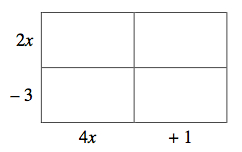Home > INT2 > Chapter 4 > Lesson 4.1.1 > Problem4-6

4-6.

Multiply the expressions below using an area model. Then verify Casey’s pattern (that the product of one diagonal equals the product of the other diagonal). Homework Help ✎

1. $(2x-3)(4x+1)$2. $(4x-8)^2$

$(4x−8)^2=(4x−8)(4x−8)$